# How many 2

How many three lettered words can be formed from letters A B C D E G H if repeats are:
a) not allowed
b) allowed

Correct result:

n1 =  210
n2 =  343

#### Solution: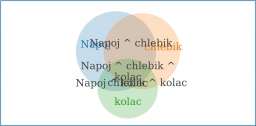We would be pleased if you find an error in the word problem, spelling mistakes, or inaccuracies and send it to us. Thank you!Tips to related online calculators

## Next similar math problems:

• Word MATEMATIKAHow many words can be created from the word MATEMATIKA by changing the order of the letters, regardless of whether or not the words are meaningful?
• WordsHow many 3 letter "words" are possible using 14 letters of the alphabet? a) n - without repetition b) m - with repetition
• Three workplacesHow many ways can we divide nine workers into three workplaces if they need four workers in the first workplace, 3 in the second workplace and 2 in the third?
• DigitsHow many natural numbers greater than 4000 which are formed from the numbers 0,1,3,7,9 with the figures not repeated, B) How many will the number of natural numbers less than 4000 and the numbers can be repeated?
• Possible combinations - word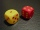How many ways can the letters F, A, I, R be arranged?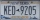A license plate has 3 letters followed by 4 numbers. Repeats are not allowed for the letters, but they are for the numbers. If they are issued at random, what is the probability that the 3 letters are in alphabetical order and the 3 numbers are consecutiv
• Three-digit numbersHow many three-digit numbers are from the numbers 0 2 4 6 8 (with/without repetition)?
• Boys and girlsThere are 11 boys and 18 girls in the classroom. Three pupils will answer. What is the probability that two boys will be among them?
• How many 4How many 4 digit numbers that are divisible by 10 can be formed from the numbers 3, 5, 7, 8, 9, 0 such that no number repeats?
• Two groupsThe group of 10 girls should be divided into two groups with at least 4 girls in each group. How many ways can this be done?
• CardsFrom a set of 32 cards we randomly pull out three cards. What is the probability that it will be seven king and ace?How many ways can we thread 4 red, 5 blue, and 6 yellow beads onto a thread?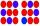How many ways can divide 10 identical candies to 5 children?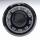A combination lock will open when the right choice of 3 numbers (from 1 to 16 inclusive) is selected. A. How many different lock combinations are possible? B. Is the combination lock named appropriately?6 purses 9 flaps 12 straps Every combination must include 1 purse, 1 flap, and 1 strap. How many are possible combinations?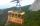Uphill leads 2 paths and 1 lift. a) How many options back and forth are there? b) How many options to get there and back by not same path are there? c) How many options back and forth are there that we go at least once a lift?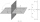There are n points, of which no three lie on one line and no four lies on one plane. How many planes can be guided by these points? How many planes are there if there are five times more than the given points?